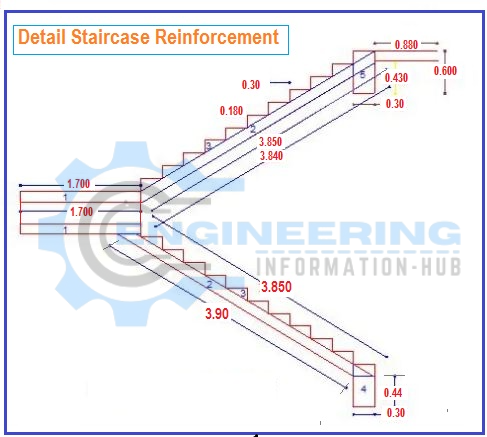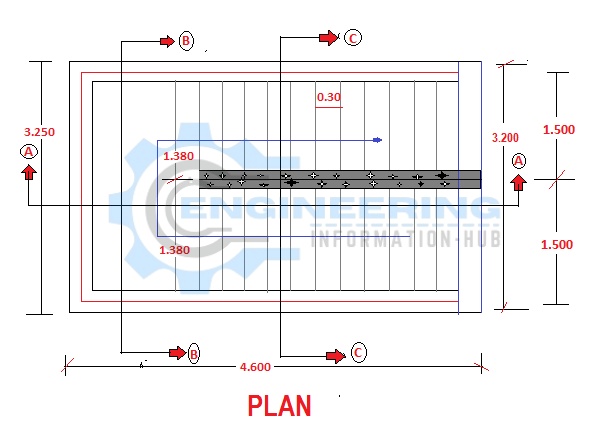Bar Bending Schedule

# Bar Bending Schedule For The Staircase

Today In this article I will explain How To Calculate The Bar Bending Schedule For The Staircase | The meaning of the Bar Bending Schedule is the Detail of steel used in the staircase.

### Bar Bending Schedule for Staircase

Given Data### Diameter of Distribution bar of Landing=8mm

Total length = 3.250m

Length of landing =1.700m

Width of Landing=1.500m

Spacing =120mm

Concrete cover=12.5mm

### Solution

Length of Distribution Bar=Total Length  – 2 Concrete cover

Length of Distribution Bar=3.250-2(12.5) =3.200m

Numbers of Distribution Bar=Total width  /spacing+1

Numbers of Distribution Bar=1500/120 +1

Numbers of Distribution Bar=12.5+1=13.5 says 14 No’s

Total Number of Distribution Bar = Bottom distribution Bar + Top distribution Bar

Total Number of Distribution Bar =14+14=28 No’s

Total Length of Distribution Bar= Length of one bar x No’s of bar

Total Length of Distribution Bar=3.200 x 28 =89.6m

### Weight Of Distribution bar for Landing

Weight of Distribution bar= d²/162.25 x total length

Weight of Distribution bar=(8)²/162.25 x 89.6

Weight of Distribution bar=64/162 x 89.6=35.34kg says 36kg

### 2-Waist slab### Given data

Diameter of Distribution bar = 8mm

Length of waist slab=3.890m+

Width of waist slab = 1.500m

Spacing of Distribution bar=140mm

### Solution

Length of Distribution bar= width of the slab

Length of Distribution bar=1.500m

Numbers of Distribution Bar= length of Waist slab/spacing +1

Numbers of Distribution Bar=3890/140+1

Numbers of Distribution Bar=27.78+1= 28.78 No’s says 29 Numbers

Total Number of Distribution Bar = Distribution Bar bottom+ Distribution Bartop

Total Number of Distribution Bar =29+29=58No’s

Total Length of Distribution Bar = Length of one bar x No’s of Bar

Total Length of Distribution Bar =1.500 x 58= 87m

### Weight of Distribution Bar for Waist Slab

Weight of Distribution Bar= d²/162.25 x total length of bar

Weight of Distribution Bar= (8)²/162.25 x 87

Weight of Distribution Bar=64/162.25 x 87 =34.31kg says 35kg

### Given data

Diameter of main bar=10mm

Spacing =80mm

Length of waist slab= L.W = 3.890m

Height of Riser= R.H=180mm

Width of landing=W.L=1.500

Width of bottom beam=W.B=0.300m

Height of bottom beam=H.B= 0.440M

### Solution

Length of main bottom bar =L.W+W.L+W.B/2 + H.B+ diameter of bar –R.H

Length of main bottom bar = 3.890+1.500+0.300/2+0.440+0.010-0.180

Length of main bottom bar =5.81m

Number of main bottom bar= Total width of slab/ spacing +1

Number of main bottom bar= 1500/80+1 =19.75=20 No’s

The total length of the main bottom bar = length of one bar x number of bar

Total length of main bottom bar =5.81 x 20 =116.2m

### Weight of Main Bottom Bar

Weight of main bottom bar =d²/162.25 x total length

Weight of main bottom bar = (10)²/162.25 x 116.200

Weight of main bottom bar =100/162.25 x 116.200 =71.61kg =72kg

### Given data

Diameter of main bar=10mm

Spacing =80mm

Length of waist slab= L.W = 3.890m

Height of Riser= R.H=180mm

Length of landing=L.L=1.500

Width of bottom beam=W.B=0.300m

Height of bottom beam=H.B= 0.440M

### Solution

Length of Top Main Bar= L.W + L.L + H.B + Diameter of bar +(W.B-2C.C)-R.H

Length of Top Main Bar=3.890+1.7+0.440+0.010+(0.300-2(12.5)-0.180

Length of Top Main Bar=6.11m

Number of Top Main Bar = Total width of slab/spacing +1

Number of Top Main Bar =1500/80+1=19.75=20No’s

The total length of the top main bar= length of one bar x No’s of bar

Total length of top main bar=6.11 x 20 =122.200m

### Weight of Top Main Bar

Weight of Top Main Bar= d²/162.25 x total length

Weight of Top Main Bar = (10)²/162.25 x 122.20

Weight of Top Main Bar = 100/162.25 x 122.20 75.315kg= 76kg

Now

## 1-Waist slab

### Given data

Diameter of Distribution bar = 8mm

Length of waist slab=3.830m+

Width of waist slab = 1.500m

Spacing of Distribution bar=140mm

### Solution

Length of Distribution bar= width of the slab

Length of Distribution bar=1.500m

Numbers of Distribution Bar= length of Waist slab/spacing +1

Numbers of Distribution Bar=3830/140+1

Numbers of Distribution Bar=27.35+1= 28.35 No’s says 29 Numbers

Total Number of Distribution Bar = Distribution Bar bottom+ Distribution Bartop

Total Number of Distribution Bar =29+29=58No’s

Total Length of Distribution Bar = Length of one bar x No’s of Bar

Total Length of Distribution Bar =1.500 x 58= 87m

### Weight of Distribution Bar for Waist Slab

Weight of Distribution Bar= d²/162.25 x total length of bar

Weight of Distribution Bar= (8)²/162.25 x 87

Weight of Distribution Bar=64/162.25 x 87 =34.31kg says 35kg

### Given data

Diameter of main bar=10mm

Spacing =80mm

Length of waist slab= L.W = 3.830m

Height of Riser= R.H=180mm

Width of landing=W.L=1.500

Width of bottom beam=W.B=0.300m

Height of bottom beam=H.B= 0.440M

### Solution

Length of main bottom bar =L.W+W.L+W.B/2 + H.B+ diameter of bar –R.H

Length of main bottom bar = 3.830+1.500+0.300/2+0.440+0.010-0.180

Length of main bottom bar =5.750m

Number of main bottom bar= Total width of slab/ spacing +1

Number of main bottom bar= 1500/80+1 =19.75=20 No’s

The total length of the main bottom bar = length of one bar x number of bar

Total length of main bottom bar =5.750 x 20 =115m

### Weight of Main Bottom Bar

Weight of main bottom bar =d²/162.25 x total length

Weight of main bottom bar = (10)²/162.25 x 115

Weight of main bottom bar =100/162.25 x 115 =70.878kg =71kg

### Given data

Diameter of main bar=10mm

Spacing =80mm

Length of waist slab= L.W = 3.830m

Height of Riser= R.H=180mm

Length of landing=L.L=1.500

Width of bottom beam=W.B=0.300m

Height of bottom beam=H.B= 0.440M

### Solution

Length of Top Main Bar= L.W + L.L + H.B + Diameter of bar +(W.B-2C.C)-R.H

Length of Top Main Bar=3.830+1.7+0.440+0.010+(0.300-2(12.5)-0.180

Length of Top Main Bar=6.050m

Number of Top Main Bar = Total width of slab/spacing +1

Number of Top Main Bar =1500/80+1=19.75=20No’s

The total length of the top main bar= length of one bar x No’s of bar

Total length of top main bar=6.050 x 20 =121m

### Weight of Top Main Bar

Weight of Top Main Bar= d²/162.25 x total length

Weight of Top Main Bar = (10)²/162.25 x 121

Weight of Top Main Bar = 100/162.25 x 121= 74.576kg= 75kg

Now

### Given data

Diameter of Ring= 8mm

Diameter of bar= 12mm

Spacing=200mm

Length of column =1.5m

Height of Column= 600mm

Concrete cover of column =25mm

Breath of column=300mm

### Solution

Length of main bar = length of column – 2c.c +2 bends(19d) -2dia of bar

Length of main bar =1.5-2(0.025) + 2(19×12)-2(12)

Length of main bar =1.5.-0.050+0.456-0.024=1.882m

Number of main bar= total length / spacing +1

Number of main bar = 1500/200+1=7.5+1=8.5= 9 no’s

The total length of the main bar= length of one bar x No’s of bar

Total length of main bar=1.882 x 9 =16.938m

### Wight of the main Bar

Wight of main bar = d²/162.25 x total length

Wight of main bar = (12)²/162.25 x 16.938

Wight of main bar= 144/162.25 x 16.938= 15.03kg =15kg

### Ring of column

Length of ring = 2 length +2 breath +2 hooks -3 diameter of bar – 4c.c

Length of ring =2(600) + 2(300)+2(9d)-3(8)-4(25)=1820mm=1.820m

Number of rings= total length /spacing +1

Number of ring = 1500/140+1=11.71=12 No’s

The total length of ring= length of one ring x no’s of ring

Total length of ring = 1.820 x 12 =21.84m

### Weight of ring

Weight of ring = d² /162.25 x total length

Weight of ring = (8)²/162.25 x 21.84 =8.61kg = 9 kg

### Total weight of 8mm bar

1st step = 36+35

2nd step= 35

Column =9

Total 36+35+9= 80kg

Total weight of 10mm bar

1st step =72+76 =148kg

2nd step = 71+75=146kh

Total =148+146= 294kg

Total weight of 12 mm bar

Column bar 12mm = 15kg

THANKS

### Raja Numan

Hi, My name is Engr. Raja Numan author of Engineering Information Hub and I am a Civil Engineer by Profession and I've specialized in the field of Quantity Surveying, Land Surveying as QC Engineer in national and multinational companies of Pakistan & Saudi Arabia.

### Related Articles

1.alisa says: